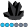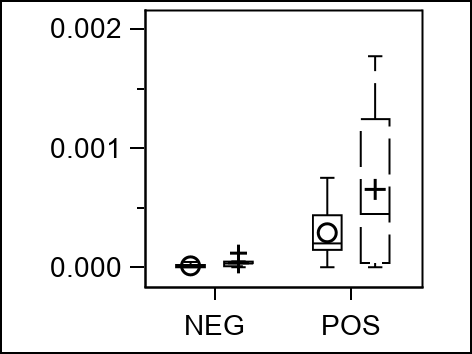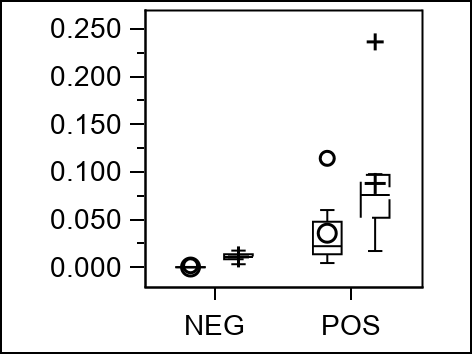das
Obsidian | Level 7

## Can I produce graphs with uniform wall dimension using sgplot and a by statement?

Can I use sgplot with a by statement to produce multiple separate graphs that have the same wall dimension, varying overall image width-heigth as necessary?

This is desired because I want the wall (or plot) area to be the same for graphs that have different y-scaling. My output has six graphs, one of which has much different y-scaling so that the y-axis numbers are to four decimal places whereas the others are just two. This causes the wall dimension of the one graph to shrink in width in order to accommodate the extra space needed by the y-axis values within the total image width.

Following is the current code where i control overall image size in the ods graphics statement, and two graphs representing the problem (same image width but different wall width; i want to preserve the latter):

``````ods _all_ close;
ods listing	style=journal3
image_DPI=300
gpath="C:\Users\das\Desktop\SAStemp\PG boxplots"
;
ods graphics on	/	width=40mm
outputfmt=tiff
imagename="listJ3"
scale=on
;
options nobyline;
proc sgplot data=paige_long
noautolegend
nowall
aspect=1
;
by marker ;
vbox pr / group=species category=movip connect=mean spread nofill;
xaxis display=(nolabel);
yaxis display=(nolabel) min=0;
run;

ods listing close ;
ods html;
quit;
``````1 ACCEPTED SOLUTION

Accepted Solutions

## Re: Can I produce graphs with uniform wall dimension using sgplot and a by statement?

I would start with something simple such as adding a VALUESFORMAT=F5.3. See if that forces all the yaxis labels to 3 decimal points.

The specific value you show means that an F4.2 might do odd things to force a second tick label, possibly resulting in multiple 0.00 tickmarks. I think that F5.3 would end up with 0.000, 0.001, 0.002 but can't test that without your data.

3 REPLIES 3

## Re: Can I produce graphs with uniform wall dimension using sgplot and a by statement?

I would start with something simple such as adding a VALUESFORMAT=F5.3. See if that forces all the yaxis labels to 3 decimal points.

The specific value you show means that an F4.2 might do odd things to force a second tick label, possibly resulting in multiple 0.00 tickmarks. I think that F5.3 would end up with 0.000, 0.001, 0.002 but can't test that without your data.das
Obsidian | Level 7

## Re: Can I produce graphs with uniform wall dimension using sgplot and a by statement?

Thank you ballardw. I think that is working well enough. I think I am trying to do to much with the by statement, as well. So my new code and the same two examples are posted below. I think this formatting suggestion combined with creating each plot separately so as to fine tune each y-axis most closely is the result that I was hoping for. Best wishes and Thank you!

``````title; footnote;
ods _all_ close;
ods listing	style=journal3
image_DPI=300
gpath="C:\Users\das\Desktop\SAStemp\PG boxplots"
;
ods graphics on	/	width=40mm
outputfmt=png
imagename="listJ3"
scale=on
;
proc sort data=paige_long;
by marker;
run;
proc sgplot data=paige_long
noautolegend
nowall
aspect=1
;
where marker = "CD3";
vbox pr / group=species category=movip spread nofill;
xaxis display=(nolabel);
yaxis display=(nolabel) min=0 VALUESFORMAT=F5.3 minor ;
run;
proc sgplot data=paige_long
noautolegend
nowall
aspect=1
;
where marker = "CD20";
vbox pr / group=species category=movip spread nofill;
xaxis display=(nolabel);
yaxis display=(nolabel) min=0 VALUESFORMAT=F5.3 minor ;
run;
proc sgplot data=paige_long
noautolegend
nowall
aspect=1
;
where marker = "CD79a";
vbox pr / group=species category=movip spread nofill;
xaxis display=(nolabel);
yaxis display=(nolabel) valeus=(0 to 0.0025 by 0.001) VALUESFORMAT=F5.3 minor;
run;
proc sgplot data=paige_long
noautolegend
nowall
aspect=1
;
where marker = "CD163";
vbox pr / group=species category=movip spread nofill;
xaxis display=(nolabel);
yaxis display=(nolabel) min=0 VALUESFORMAT=F5.3 minor ;
run;
proc sgplot data=paige_long
noautolegend
nowall
aspect=1
;
where marker = "Iba1";
vbox pr / group=species category=movip spread nofill;
xaxis display=(nolabel);
yaxis display=(nolabel) min=0 VALUESFORMAT=F5.3 minor ;
run;
proc sgplot data=paige_long
noautolegend
nowall
aspect=1
;
where marker = "IL-17";
vbox pr / group=species category=movip spread nofill;
xaxis display=(nolabel);
yaxis display=(nolabel) min=0 VALUESFORMAT=F5.3 minor ;
run;

ods listing close ;
ods html;
quit;
``````## Re: Can I produce graphs with uniform wall dimension using sgplot and a by statement?

Hi,

Have you tried this in your sgplot statement?

uniform=yscale

Just a thought 🙂

Discussion stats
• 3 replies
• 729 views
• 0 likes
• 3 in conversation